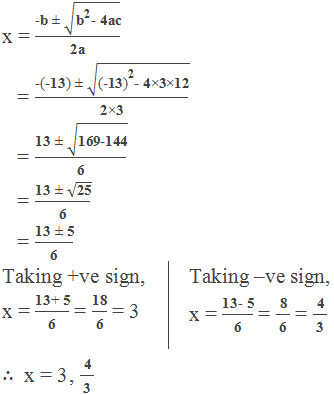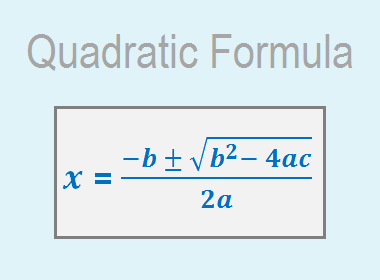A formula to calculate the roots or solutions of a quadratic equation ax2 + bx + c = 0 is known as the Quadratic Formula which is given as,where a is the coefficient of x2, b is the coefficient of x and c is the constant term. We use this formula to find the required solutions (roots) of the given quadratic equation.

Some special conditions:

When b2 > 4ac, there are two distinct real roots.

When b2 = 4ac, there is a single real root.

When b2 < 4ac, there is no real roots.

**********************

10 Math Problems officially announces the release of Quick Math Solver, an android APP on the Google Play Store for students around the world.**********************

ax2 + bx + c = 0, where a, b and c are constants and a≠ 0.

Rewriting the given equation as

ax2 + bx = – c

Dividing both sides by the coefficient of x2 i.e. a we get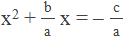In order to make the left-hand side a perfect square, we take half the coefficient of x,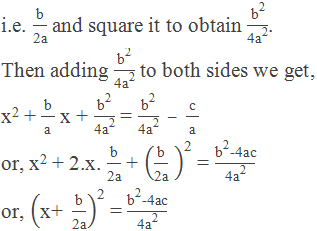Taking square root to both sides, we get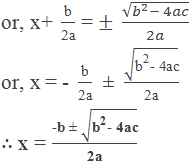Hence the Quadratic Formula is derived.

### Workout Examples

Example 1: Solve x2 – 7x + 12 = 0 by using quadratic formula.

Solution: Here,

x2 – 7x + 12 = 0

Comparing this equation with ax2 + bx + c = 0 we get,

a = 1

b = –7

c = 12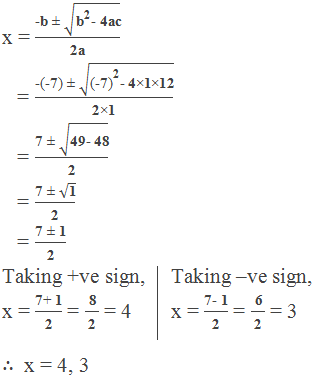Example 2: Solve (p – q)x2 + (p + q)x + 2q = 0 by using quadratic formula.

Solution: Here,

(p – q)x2 + (p + q)x + 2q = 0

Comparing this equation with ax2 + bx + c = 0 we get,

a = (p – q)

b = (p + q)

c = 2q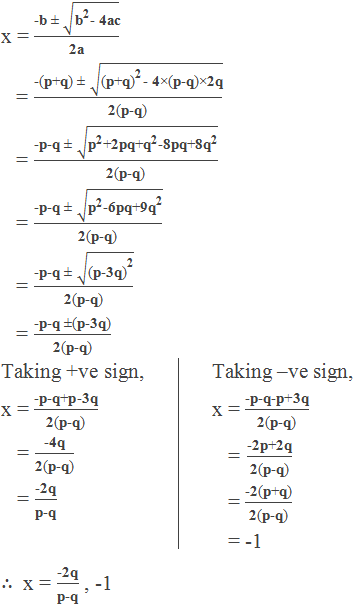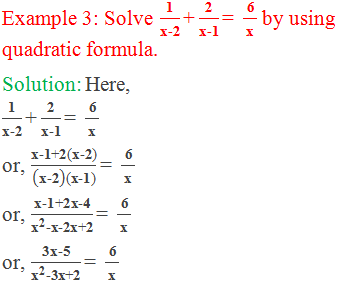or, 6x2-18x+12 = 3x2-5x

or, 6x2–18x+12–3x2+5x = 0

or, 3x2–13x+12= 0

Comparing this equation with ax2 + bx + c = 0 we get,

a = 3

b = –13

c = 12